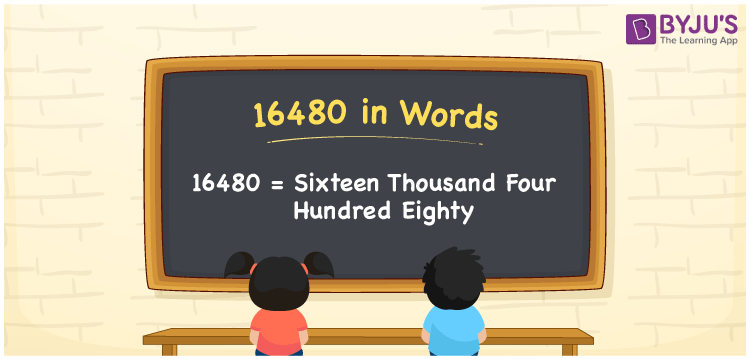# 16480 in Words

16480 in words is written as Sixteen thousand four hundred eighty In both the International System of Numerals and the Indian System of Numerals, 16480 is written as Sixteen thousand four hundred eighty The number 16480 is a Cardinal Number as it denotes some quantity. For example, “the tablet costs 16480 rupees”.

 16480 in Words Sixteen thousand four hundred eighty Sixteen thousand four hundred eighty in Number 16480

## 16480 in English Words

16480 in English words is read as “Sixteen thousand four hundred eighty”.## How to Write 16480 in Words?

To write 16480 in words, we shall use the place value chart. In the place value chart, put 1 in the ten thousands, 6 in the thousands, 4 in the hundreds, 8 in the tens and 0 in the ones. Let us make a place value chart to write the number 16480 in words.

 Ten Thousands Thousands Hundreds Tens Ones 1 6 4 8 0

Thus, we can write the expanded form as

1 × Ten Thousand + 6 × Thousand + 4 × Hundred + 8 × Ten + 0 × One

= 1 × 10000 + 6 × 1000 + 4 × 100 + 8 × 10 + 0 × 1

= 10000 + 6000 + 400 + 80 + 0

= 16480

= Sixteen thousand four hundred eighty

16480 is a natural number, the successor of 16479 and the predecessor of 16481.

16480 in words – Sixteen thousand four hundred eighty

• Is 16480 an odd number? – No
• Is 16480 an even number? – Yes
• Is 16480 a perfect square number? – No
• Is 16480 a perfect cube number? – No
• Is 16480 a prime number? – No
• Is 16480 a composite number? – Yes

## Frequently Asked Questions on 16480 in Words

Q1

### How to write 16480 in words?

16480 in words is written as Sixteen thousand four hundred eighty.
Q2

### How to write 16480 in the International and Indian System of Numerals?

In both, the system of numerals, 16480 in words, is written as Sixteen thousand four hundred eighty.
Q3

### How to write 16480 in a place value chart?

In the place value chart, write 1 in the ten thousands, 6 in the thousands, 4 in the hundreds, 8 in the tens and 0 in the ones, respectively.